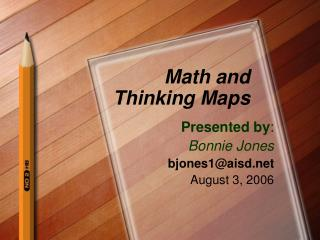DownloadDownload PresentationMath and Thinking Maps

# Math and Thinking Maps

Download Presentation## Math and Thinking Maps

- - - - - - - - - - - - - - - - - - - - - - - - - - - E N D - - - - - - - - - - - - - - - - - - - - - - - - - - -
##### Presentation Transcript

1. Math and Thinking Maps Presented by: Bonnie Jones bjones1@aisd.net August 3, 2006

2. What do you hope to walk away with today? Why did you choose this session?

3. Thinking and Math What type of thinking occurs daily in math? Thinking done in math class

4. Thinking maps represent 8 thought processes. Look at your list of thinking…match maps to the thinking that students do in math class. Thinking in Math Class Circle Tree

5. Activities--Circle Map-brainstorming, defining *What do you know about ______ ? *

6. Activities-Double Bubble Map-like a Venn diagram--compare/contrast *Greatest Common Factor/Least Common Multiple *compare geometric figures/shapes *compare terms: perimeter/area

7. Activities-Tree Map-categorize, sort, organize *Sorting--measurement activity--in., ft., yd. *Sort: geometric figures/shapes *Sort word problems: add, subtract, multiply, divide, multi-step *Sort: prime numbers, composite numbers, odd numbers, even numbers **Remember: pictures, words, or both

8. Activities-Brace Map-analyze, break into parts

9. Activities-Brace Map-analyze, break into parts

10. Activities-Brace Map-analyze, break into parts 18. When Maggie went to her sister’s graduation, she saw that 300 students were graduating. Maggie noticed that equal numbers of graduating students were seated in 5 different sections of the auditorium. How many graduating students were seated in 1 section? From: www.tea.state.tx.us 4th gr math 2004

11. Activities-Brace Map-analyze, break into parts When Maggie went to her sister’s graduation, she saw that 300 students were graduating. Maggie noticed that equal numbers of graduating students were seated in 5 different sections of the auditorium. How many graduating students were seated in 1 section?

12. Activities-Brace Map-analyze, break into parts 300 students equal numbers 5 different sections How many graduating students were seated in 1 section?

13. Activities-Brace Map-analyze, break into parts 300 divided by 5 equal parts =_______

14. Activities-Brace Map-analyze, break into parts

15. Activities-Flow Map--Sequence Have students break down the steps in answering math problems… What do you do first, second, third, and so forth…

16. Activities-Multi-flow Map--cause/effect 48 *1 Making 48 56-8 32 + 16 96/2

17. Activities-Bridge Map--Analogy/relationships 19. Hal used this table to find the total prices of different numbers of tickets to a basketball game. (Table is included in the actual problem…I put the info in a bridge map). 20 10 15 5 \$15 \$45 \$30 \$60 What is the RELATING FACTOR? Based on the information in the table, how would Hal find the price of 1 ticket? A Multiply the number of tickets by 5 B Add 3 to the number of tickets C Subtract the number of tickets from the price of tickets D Divide the price of tickets by the number of tickets From: www.tea.state.tx.us 4th gr math 2004

18. Activities-Bridge Map--Analogy/relationships

19. Your turn…… With a partner: *Decide on a math concept you might teach or a problem a student might encounter *What thinking map would you model or expect students to use? *Use chart paper to make your map